﻿ 基于CFD的舵机选型方法
 舰船科学技术2018, Vol. 40Issue (4): 143-146PDF

Selection method of steering gear based on CFD
LONG Jin-jun, LI Yan
Department of Naval Architecture and Ocean Engineering,Nantong Shipping College, Nantong 226010, China
Abstract: In this paper, a new method of steering gear selection is proposed based on the analysis of the limitation of conventional steering gear selection methods. First, get rudder moment curve by CFD calculating. Then, make a series of steering torque curve under different system pressures after the rudder tiller and cylinder parameters were determined. At last, by comparing the rudder moment curve with series of steering torque curves, obtain steering torque and steering system pressure. Three parties, the ship designer, steering gear manufacturer and ship builder, are involved together in steering gear selection which is required by this method.
Key words: steering gear selection     CFD     rudder moment curve     steering torque curve
0 引　言

1 舵力数值模拟计算 1.1 舵参数介绍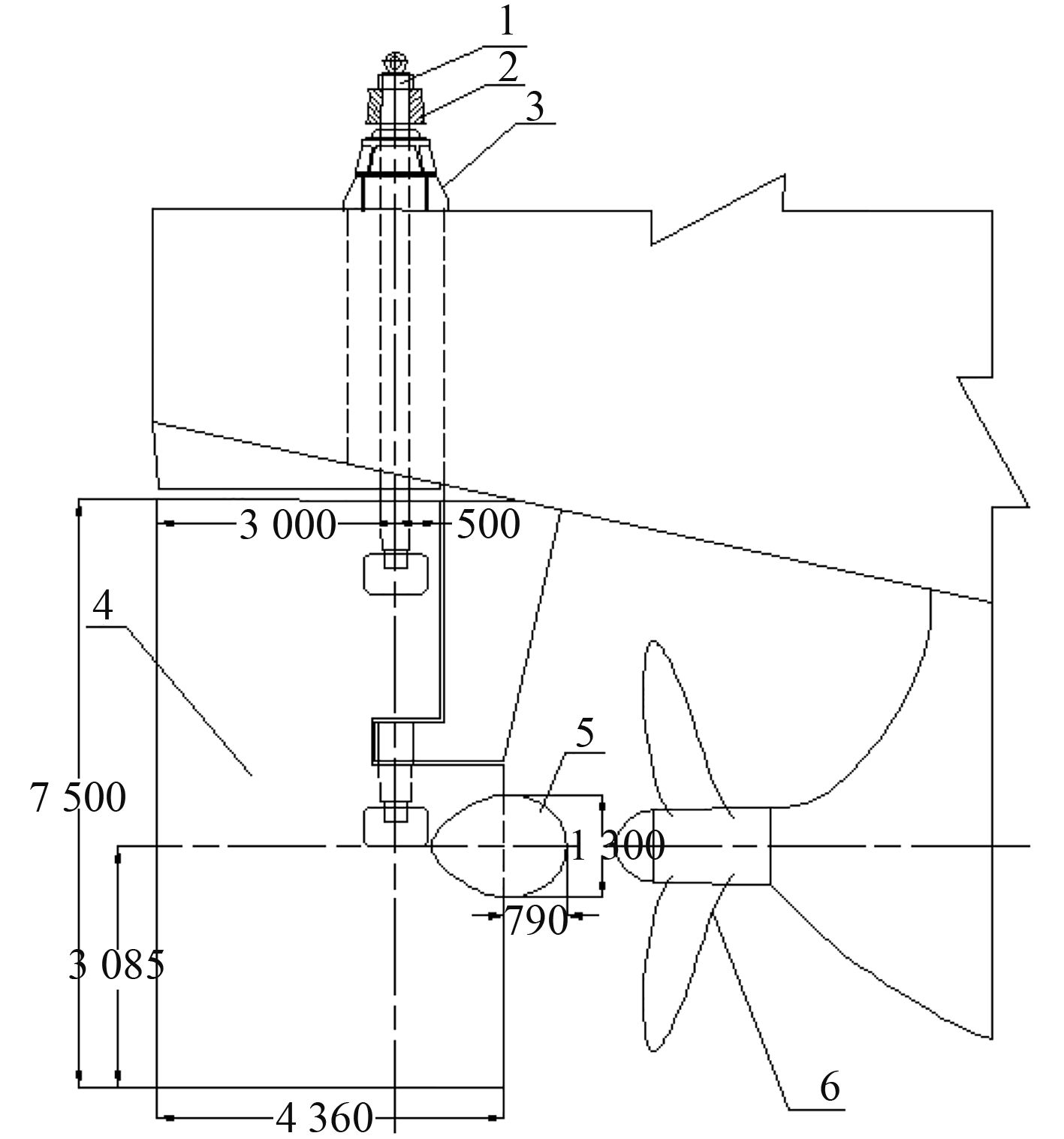图 1 舵系统组成图 Fig. 1 The composition of rudder system
1.2 利用Fluent软件计算舵力及舵杆扭矩

 ${C_L} = {P_L}/0.5\rho {\nu ^2}A\text{，}$ (1)
 ${C_D} = {P_D}/0.5\rho {\nu ^2}A\text{，}$ (2)
 ${C_X} = {X_C}/b\text{。}$ (3)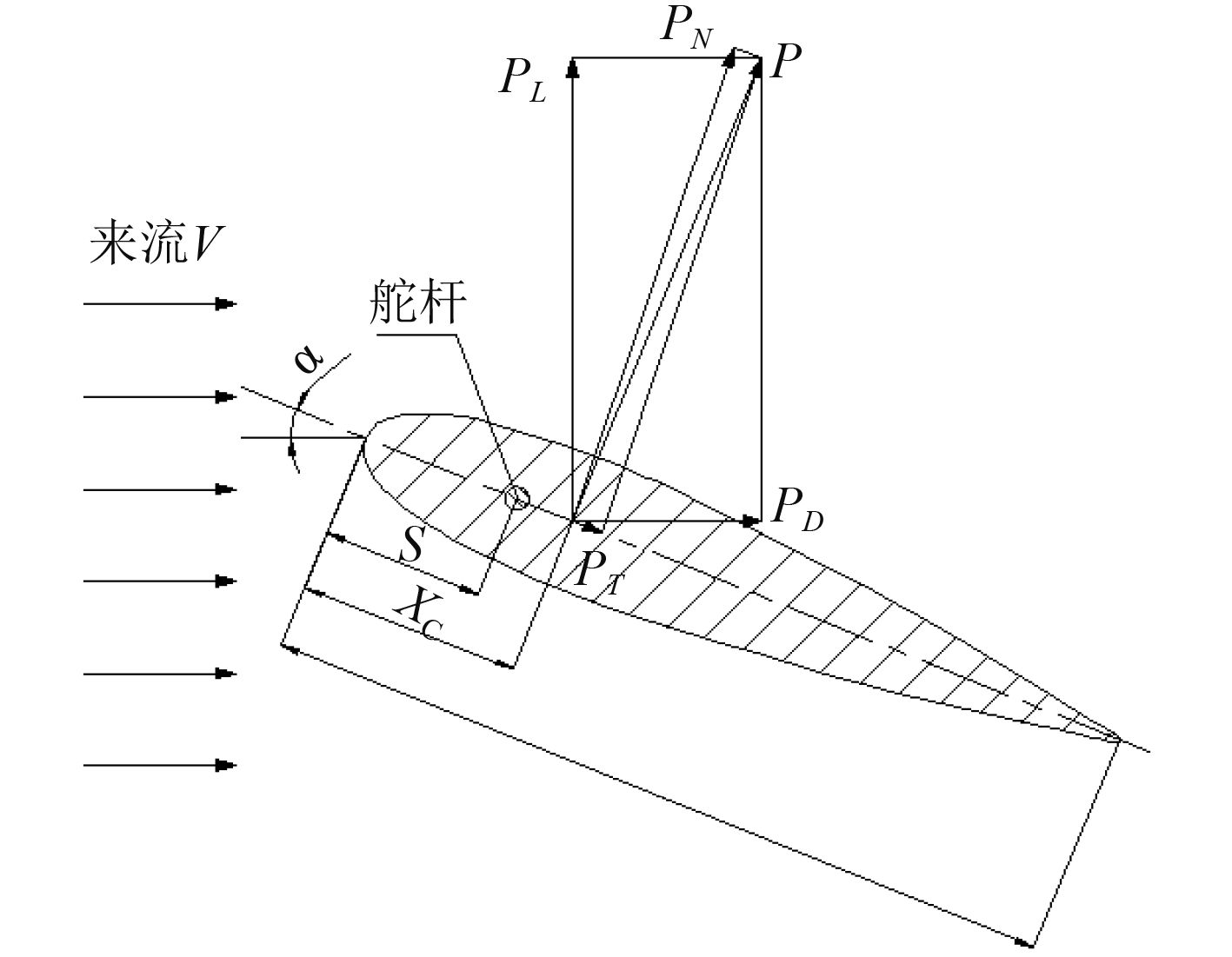图 2 舵受力图 Fig. 2 The force of rudder blade

 ${P_N} = {P_L}\cos \alpha + {P_D}\sin \alpha \text{，}$ (4)

 ${M_\alpha } = {P_N}\left( {{x_c} - s} \right)\text{。}$ (5)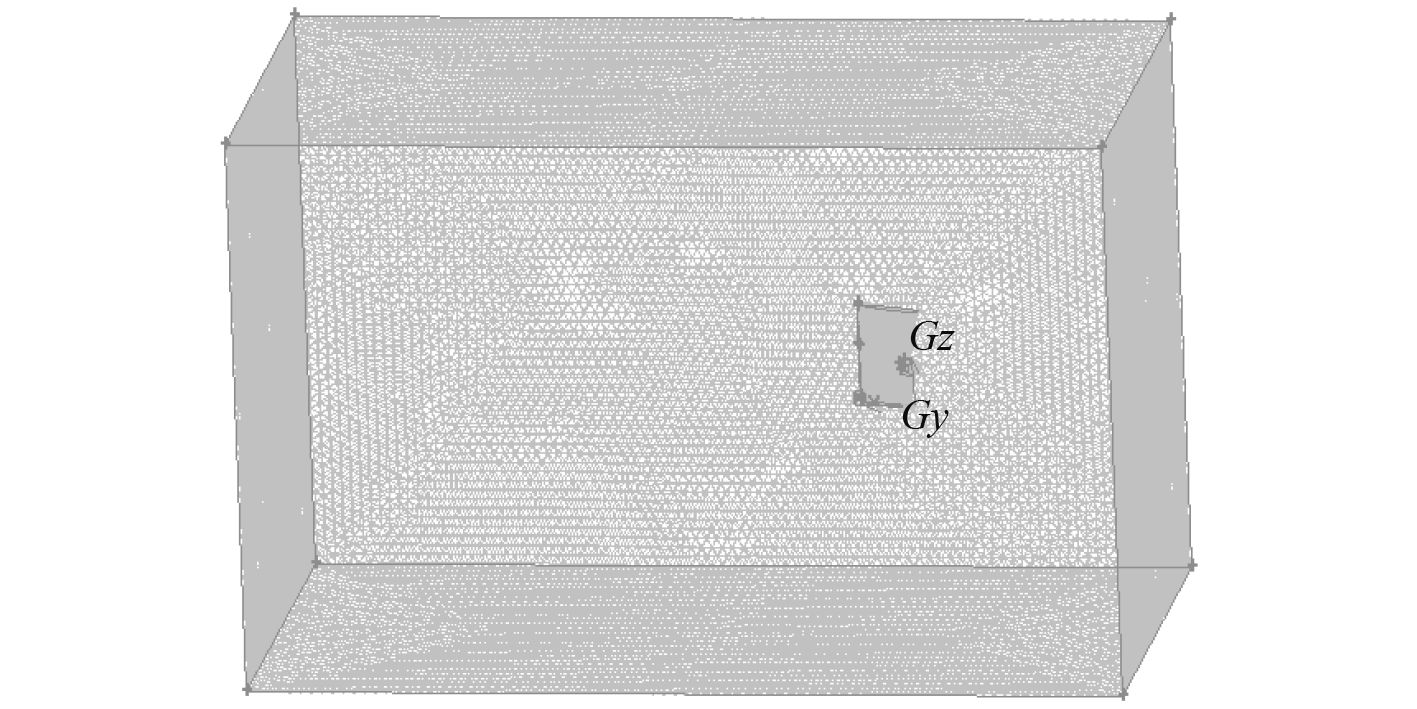图 3 计算域的网格划分 Fig. 3 Mesh generation of compute field表 1 应用Fluent软件计算的舵杆力矩值 Tab.1 rudder torque computed by fluent software
2 舵杆扭矩校核

 $F = 132{K_1}{K_2}{K_3}A{V_{\rm d}}^2\;\text{。}$ (6)

 $T = FR\text{。}$ (7)

 $R = {c_1}({\alpha _1} - {\rm{ }}{A_{1f}}/{A_1}){A_1}/A + {c_2}({\alpha _2} - {A_{2f}}/{A_2}){\rm{ }}{A_2}/A\text{。}$ (8)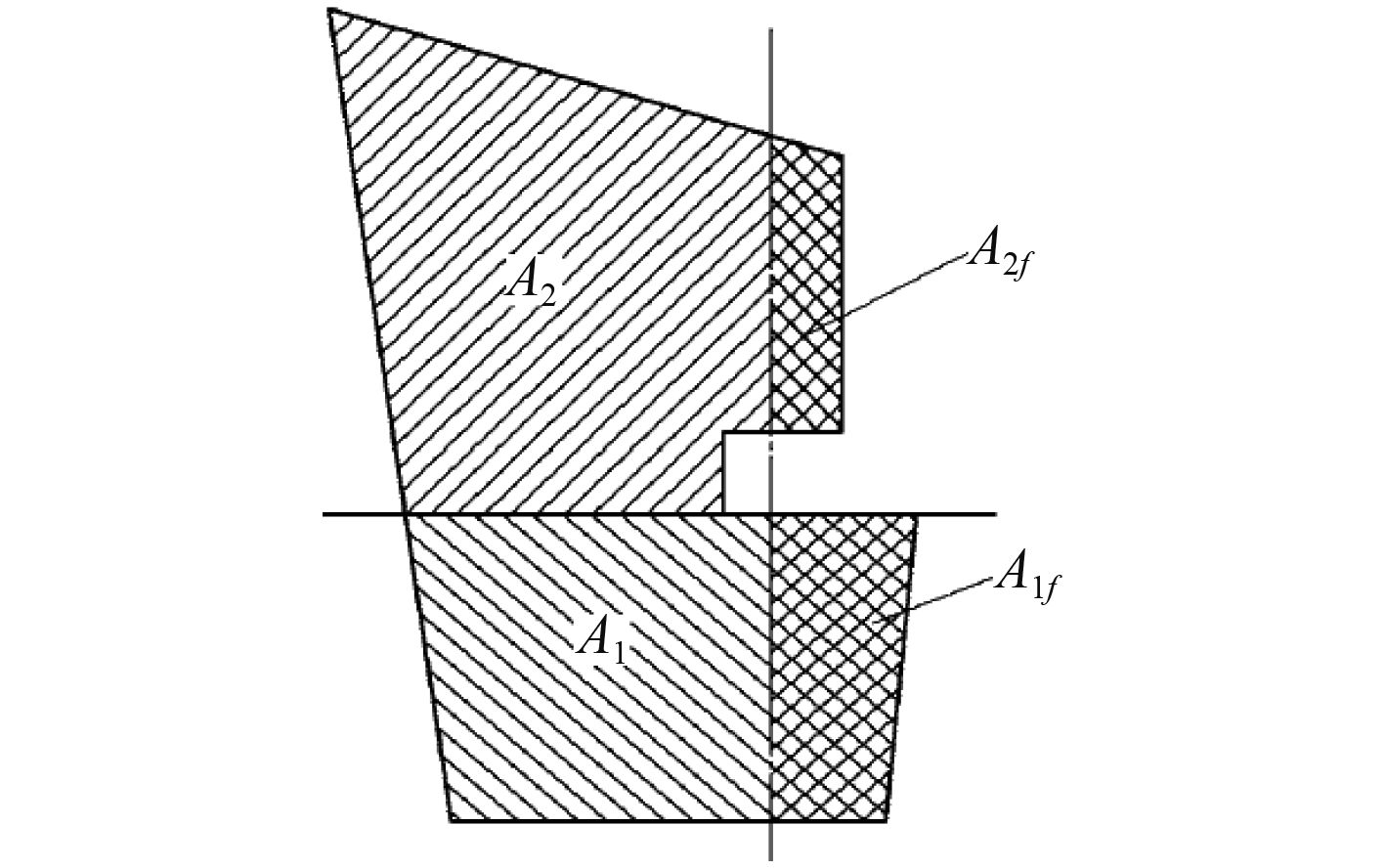图 4 规范对舵叶的划分 Fig. 4 The division of the rudder area

3 舵机选型 3.1 舵机扭矩的确定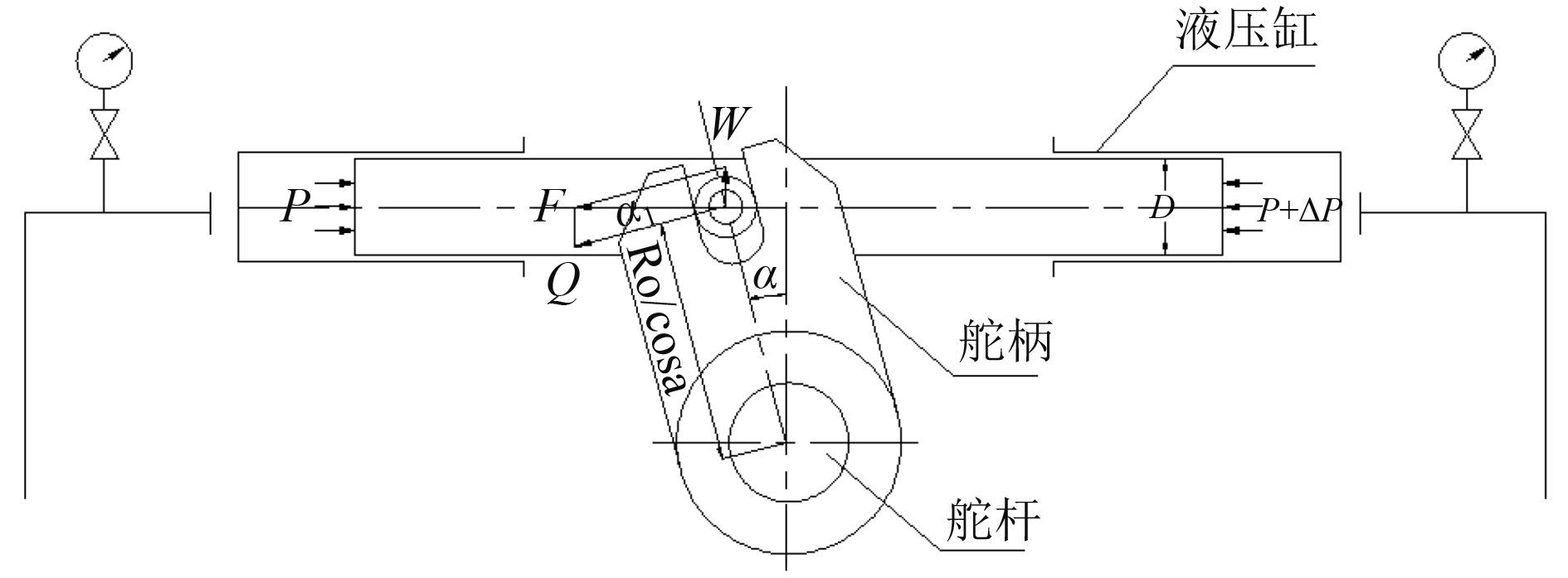图 5 舵机推舵结构受力图 Fig. 5 The force of Steering mechanism

 ${M_Q} = \left( {F/\cos \alpha } \right) \times \left( {{R_o}/\cos \alpha } \right) \times n \times \eta \times {10^6} \text{。}$ (10)表 2 舵机扭矩计算表 Tab.2 Torque calculation of the steering gear
3.2 舵机选型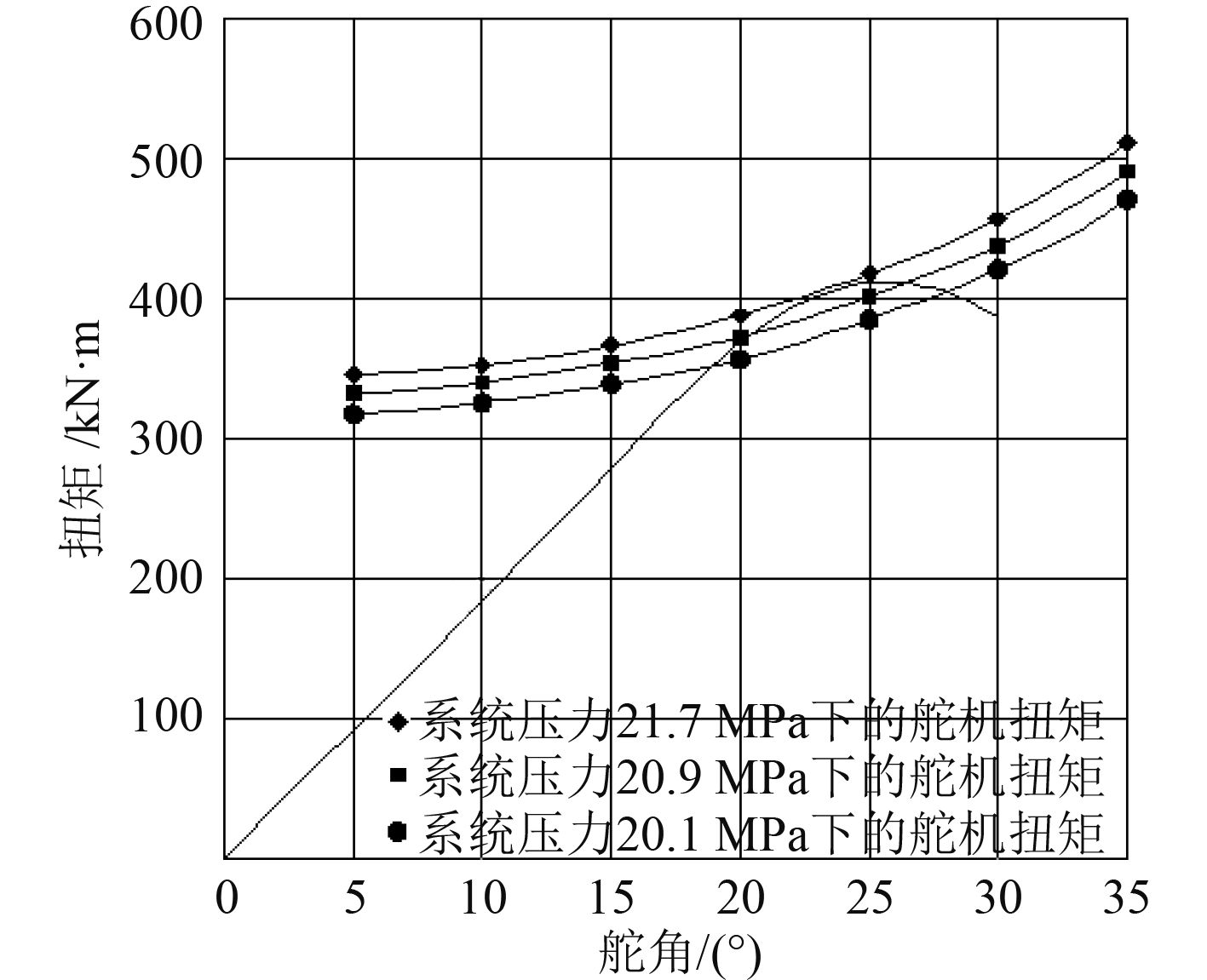图 6 舵机扭矩曲线、舵杆扭矩曲线图 Fig. 6 The steering torque curve, Rudder Torque Curve

4 结　语

  王孝霖, 许历, 韩星星, 等. 船用舵机液压系统分析及典型故障处理[J]. 机床与液压, 2015(22): 187–189.  HOU Li-xun, Wang Chao, Chang Xin, et al. Hydrodynamic performance analysis of propeller-rudder system with the rudder parameters changing[J]. Journal of Marine Science and Application, 2013(4): 406–412. https://link.springer.com/content/pdf/10.1007/s11804-013-1211-0.pdf  何苗, 王超, 郭春雨, 等. 舵球几何参数对螺旋桨水动力性能的影响[J]. 武汉理工大学学报, 2011(7): 68–72. https://www.wenkuxiazai.com/doc/112362ce9b89680203d825e6.html  程枳宁, 陈正寿, 黄聪汉, 等. 船舶附加水动力节能装置研究进展[J]. 浙江海洋学院学报(自然科学版), 2016(1): 70–75. http://www.wanfangdata.com.cn/details/detail.do?_type=perio&id=zjhyxyxb-zr201601015  周广礼, 董文才, 姚朝帮. 半悬挂舵敞水水动力性能数值计算方法研究[C]//2013年船舶水动力学学术会议论文集, 2013, 104–112.  林友红. 半悬挂舵的敞水水动力性能与舵力预估方法研究[J]. 舰船电子工程, 2015(10): 142–147.  周广礼, 姚朝帮. 小展弦比翼大攻角水动力数值计算方法[J]. 舰船科学技术, 2016(6): 337–341. http://www.cnki.com.cn/Article/CJFDTotal-HEBD201502006.htm  中国船级社. 钢质海船入级与建造规范[S]. 北京人民交通出版社, 2015.  梅琴生. 船用舵[M]. 北京: 人民交通出版社, 1981: 97–98.  苏东海, 黄鑫. 船舶舵机液压参数设计[J]. 液压与气动, 2008(6): 14–16. https://www.wenkuxiazai.com/doc/6451a0768e9951e79b892727.html  汪家政, 翟连忠. 大型船舶舵系安装精度控制关键技术研究[J]. 船舶工程, 2011(2): 71–73. http://www.wanfangdata.com.cn/details/detail.do?_type=perio&id=cbgc201102017